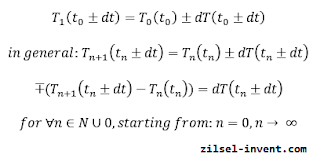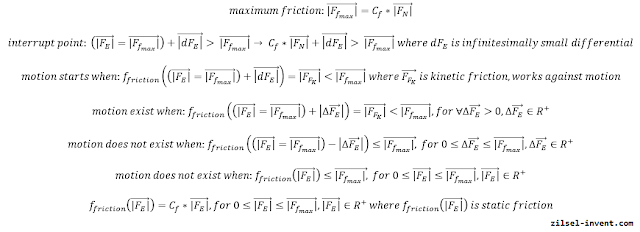### Feel the force around you – Frictional force Part I

This is the first article in a series intended to explain the force of friction. If somebody asks you: what is the force of friction? Just give a simple answer, it is a force! Maybe it sounds crazy, but it is a 100% true answer. Let’s start thinking in equations. If we do have force on the left side of an equation that also mean that we do have a force on the right side of the equation. It is not possible to have force on the left and frogs on the right, because force and frogs are not the same things by nature. Basically, what is a force of friction? is a stupid question. The better question is: which force is the force of friction?

That is a very good question. We already started a discussion about the normal force included in the frictional force calculations. Anyway, even if a normal force is used to calculate the maximum value of the frictional force, there are a lot of differences between normal and frictional force, and also similarities. The frictional force is a reaction force (acts in the opposite direction) as it is a normal force, but with different behavior. Frictional force has different behavior depending on the situation, but we will see that behavior also depends on the geometrical shape of the object. In some cases it works against motion in some cases it provides the motion. Comparing to other natural occurrences like temperature, friction is a natural phenomena. Mathematically spiking temperature is a continuous function without interrupts, like it is presented in the following equations:Continuous function in general.

𝑒𝑟𝑒 ther side, friction does have interrupt in sOn the other side frictional force (vector Ff) has interrupt in point where applied external force (vector FE) overcomes the normal force (vector FN) multiplied by a frictional force coefficient (Cf), as it is presented in the following equations:Motion descriptions relate to the sliding motion of the cubical shaped objectsThe frictional force interrupted function

By equations and the above picture, frictional force contains two domains: static friction domain and kinetic friction domain. In some cases static friction domain works against motion in some cases it provides the motion. We will see that it depends on the geometrical shape of the object. For example: for cubes (and similarly shaped objects) it works against the motion. In that case it is important for external forces to overcome the maximum frictional force. On the other side, for rounded objects like wheels static friction domain provides the motion. Unlike static friction, kinetic friction always act against the motion. Now, let’s answer the question: which force is the frictional force? Well, the frictional force is a reactional force which means that it is equal to the applied external force, it acts by Third, Newton Law and it is linear, in case when it belongs to the static friction domain. To calculate maximum static frictional resistance (force) it is necessary first to calculate a normal force, which means that first we need to know the total weight of the mobile robot including incline angle. In the next articles we will see that maximum normal force depends on inclines, which means that normal force is not a constant. When an applied external force acts in kinetic frictional domain, the frictional force is also a reaction force, but with less intensity which leads to the motion. In general, Frictional force is reactional force, like normal force is, in case when applied external force is greater than zero.

Related articles:
Feel the force around you – Normal force
Normal force - Real catalog examples and calculations
Feel the force around you – Frictional force Part II

Feel the force around you – Frictional force Part IV

Programmable autonomous vehicles – Fundamentals, Part I